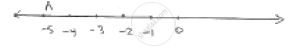# Solve the Equation 3x + 2 = X − 8, and Represent the Solution on the Number Line . - Mathematics

Solve the equation 3x + 2 = x − 8, and represent the solution on the number line .

#### Solution3x + 2 = x - 8

⇒  3x - x = 8 - 2

⇒ 2x = - 10

⇒ x = - 5

Points A represents -5 on number line

Concept: Solution of a Linear Equation
Is there an error in this question or solution?

#### APPEARS IN

RD Sharma Mathematics for Class 9
Chapter 7 Linear Equations in Two Variables
Exercise 7.4 | Q 3.1

Share